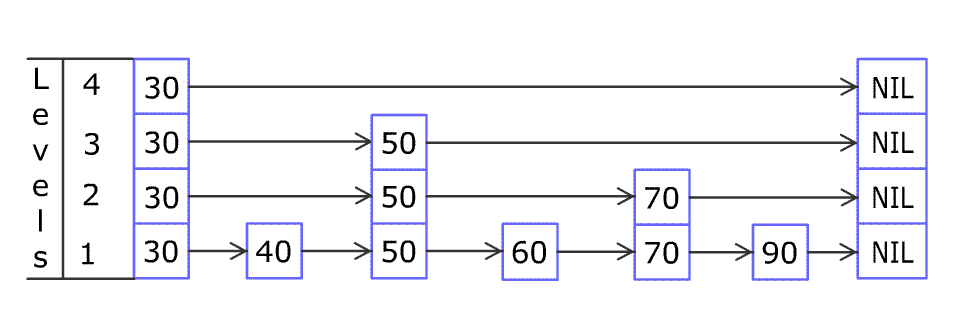## Algorithm

Problem Name: 1206. Design Skiplist

Design a Skiplist without using any built-in libraries.

A skiplist is a data structure that takes `O(log(n))` time to add, erase and search. Comparing with treap and red-black tree which has the same function and performance, the code length of Skiplist can be comparatively short and the idea behind Skiplists is just simple linked lists.

For example, we have a Skiplist containing `[30,40,50,60,70,90]` and we want to add `80` and `45` into it. The Skiplist works this way:Artyom Kalinin [CC BY-SA 3.0], via Wikimedia Commons

You can see there are many layers in the Skiplist. Each layer is a sorted linked list. With the help of the top layers, add, erase and search can be faster than `O(n)`. It can be proven that the average time complexity for each operation is `O(log(n))` and space complexity is `O(n)`.

Implement the `Skiplist` class:

• `Skiplist()` Initializes the object of the skiplist.
• `bool search(int target)` Returns `true` if the integer `target` exists in the Skiplist or `false` otherwise.
• `void add(int num)` Inserts the value `num` into the SkipList.
• `bool erase(int num)` Removes the value `num` from the Skiplist and returns `true`. If `num` does not exist in the Skiplist, do nothing and return `false`. If there exist multiple `num` values, removing any one of them is fine.

Note that duplicates may exist in the Skiplist, your code needs to handle this situation.

Example 1:

```Input
[[], , , , , , , , , ]
Output
[null, null, null, null, false, null, true, false, true, false]

Explanation
Skiplist skiplist = new Skiplist();
skiplist.search(0); // return False
skiplist.search(1); // return True
skiplist.erase(0);  // return False, 0 is not in skiplist.
skiplist.erase(1);  // return True
skiplist.search(1); // return False, 1 has already been erased.```

Constraints:

• `0 <= num, target <= 2 * 104`
• At most `5 * 104` calls will be made to `search`, `add`, and `erase`.

## Code Examples

### #1 Code Example with Java Programming

```Code - Java Programming```

``````
class Skiplist {

private Random random;

public Skiplist() {
this.random = new Random();
}

public boolean search(int target) {
while (curr != null) {
while (curr.next != null && curr.next.val < target) {
curr = curr.next;
}
if (curr.next != null && curr.next.val == target) {
return true;
}
curr = curr.down;
}
return false;
}

Stack stack = new Stack<>();
while (curr != null) {
while (curr.next != null && curr.next.val < num) {
curr = curr.next;
}
stack.push(curr);
curr = curr.down;
}
boolean newLevel = true;
Node downNode = null;
while (newLevel && !stack.isEmpty()) {
curr = stack.pop();
Node newNode = new Node(num);
newNode.next = curr.next;
newNode.down = downNode;
curr.next = newNode;
downNode = curr.next;
newLevel = random.nextDouble() < 0.5;
}
if (newLevel) {
}
}

public boolean erase(int num) {
boolean found = false;
while (curr != null) {
while (curr.next != null && curr.next.val < num) {
curr = curr.next;
}
if (curr.next != null && curr.next.val == num) {
found = true;
curr.next = curr.next.next;
}
curr = curr.down;
}
return found;
}

private static class Node {
int val;
Node next;
Node down;

public Node(int val) {
this.val = val;
}
}
}
``````
Copy The Code &

Input

cmd

Output

cmd
[null, null, null, null, false, null, true, false, true, false]

### #2 Code Example with Javascript Programming

```Code - Javascript Programming```

``````
class Skiplist {
constructor() {
this.head = { down: null, right: null, val: -Infinity }
}
search(val) {
while (curr) {
while (curr.right && curr.right.val <= val) {
curr = curr.right
}
if (curr.val == val) {
return true
}
curr = curr.down
}
return false
}
const insertion_positions = []
while (curr) {
while (curr.right && curr.right.val < val) {
curr = curr.right
}
insertion_positions.push(curr)
curr = curr.down
}
let insert = true
let down = null
while (insert && insertion_positions.length) {
const position = insertion_positions.pop()
const node = { down, val, right: position.right }
position.right = node
down = node
insert = Math.random() < 0.5
}
if (insert) {
const node = { val, down }
}
}
erase(val) {
const erase_positions = []
while (curr) {
while (curr.right && curr.right.val < val) {
curr = curr.right
}
if (curr.right && curr.right.val == val) {
erase_positions.push(curr)
}
curr = curr.down
}
const seen = erase_positions.length > 0
for (const position of erase_positions) {
position.right = position.right && position.right.right
}
return seen
}
}
``````
Copy The Code &

Input

cmd